# Difference between revisions of "Chemical potential"

## Classical thermodynamics

Definition: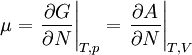$\mu=\left. \frac{\partial G}{\partial N}\right\vert_{T,p} = \left. \frac{\partial A}{\partial N}\right\vert_{T,V}$

where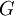$G$ is the Gibbs energy function, leading to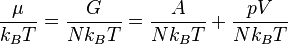$\frac{\mu}{k_B T}=\frac{G}{N k_B T}=\frac{A}{N k_B T}+\frac{p V}{N k_B T}$

where$A$ is the Helmholtz energy function,$k_B$ is the Boltzmann constant,$p$ is the pressure,$T$ is the temperature and$V$ is the volume.

## Statistical mechanics

The chemical potential is the derivative of the Helmholtz energy function with respect to the number of particles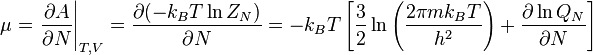$\mu= \left. \frac{\partial A}{\partial N}\right\vert_{T,V}=\frac{\partial (-k_B T \ln Z_N)}{\partial N} = - k_B T \left[ \frac{3}{2} \ln \left(\frac{2\pi m k_BT}{h^2}\right) + \frac{\partial \ln Q_N}{\partial N} \right]$

where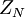$Z_N$ is the partition function for a fluid of$N$ identical particles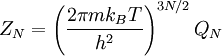$Z_N= \left( \frac{2\pi m k_BT}{h^2} \right)^{3N/2} Q_N$

and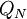$Q_N$ is the configurational integral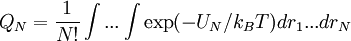$Q_N = \frac{1}{N!} \int ... \int \exp (-U_N/k_B T) dr_1...dr_N$

## Kirkwood charging formula

The Kirkwood charging formula is given by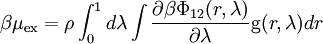$\beta \mu_{\rm ex} = \rho \int_0^1 d\lambda \int \frac{\partial \beta \Phi_{12} (r,\lambda)}{\partial \lambda} {\rm g}(r,\lambda) dr$

where$\Phi_{12}(r)$ is the intermolecular pair potential and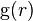${\rm g}(r)$ is the pair correlation function.